Ex 3.2

Chapter 3 Class 12 Matrices
Serial order wise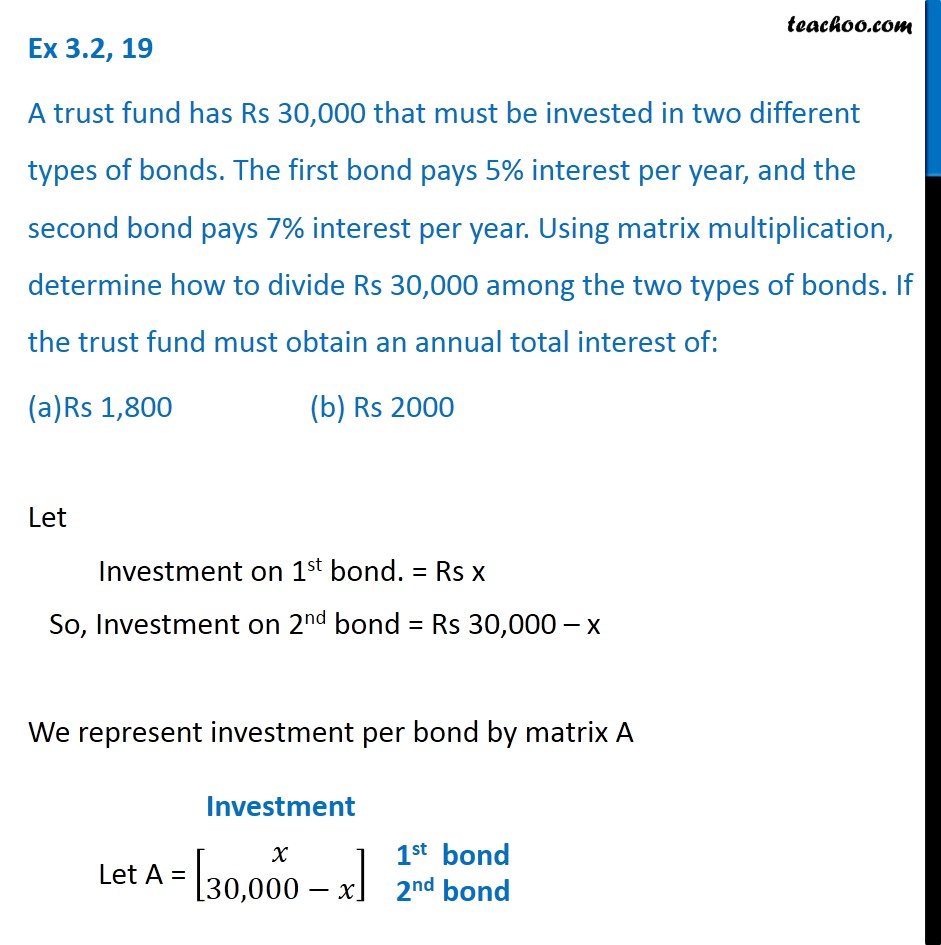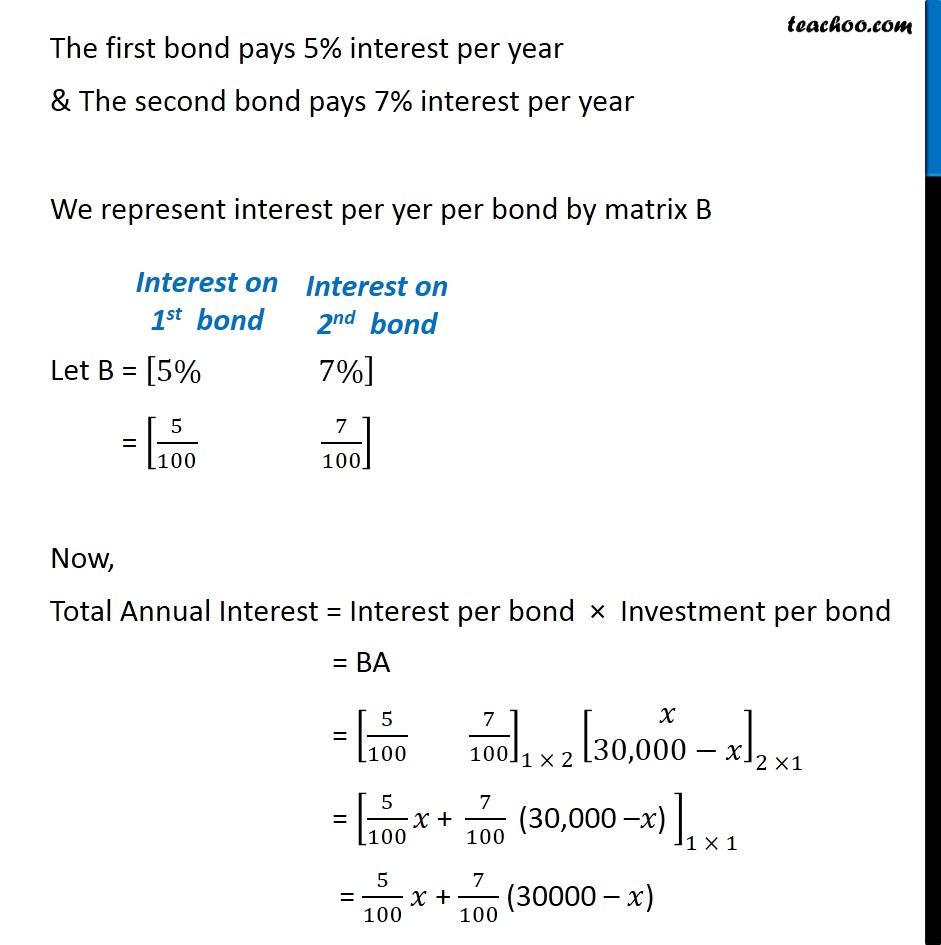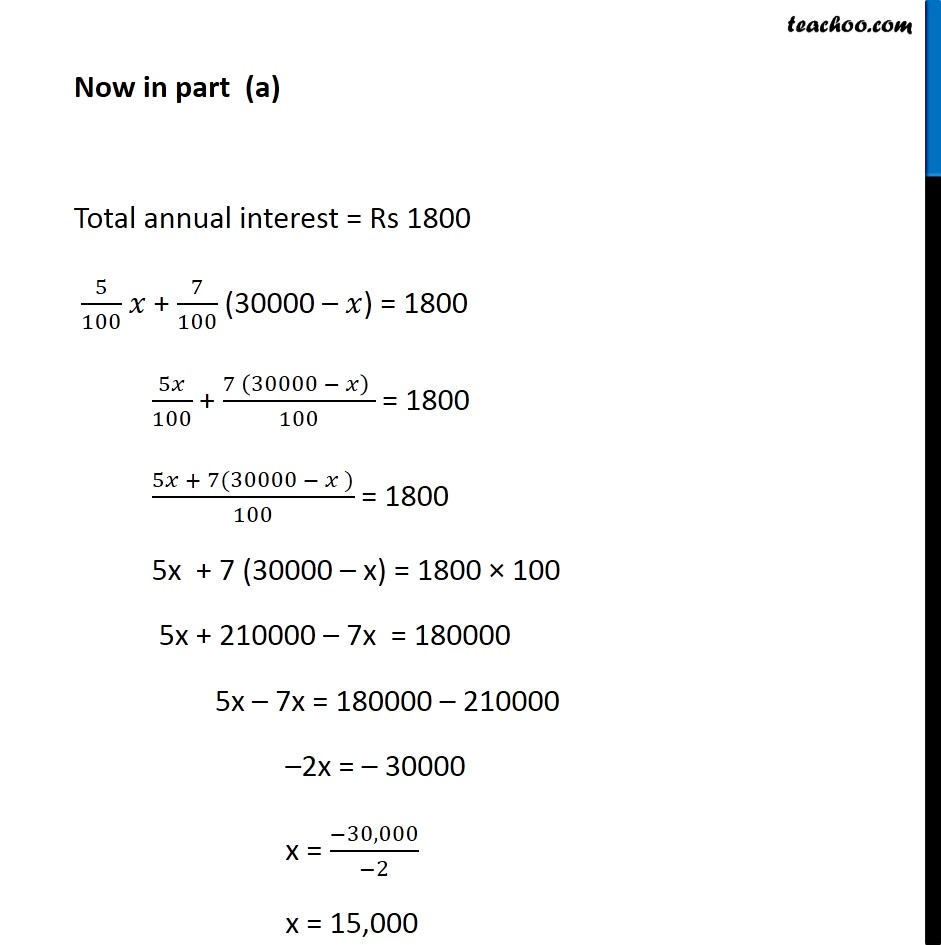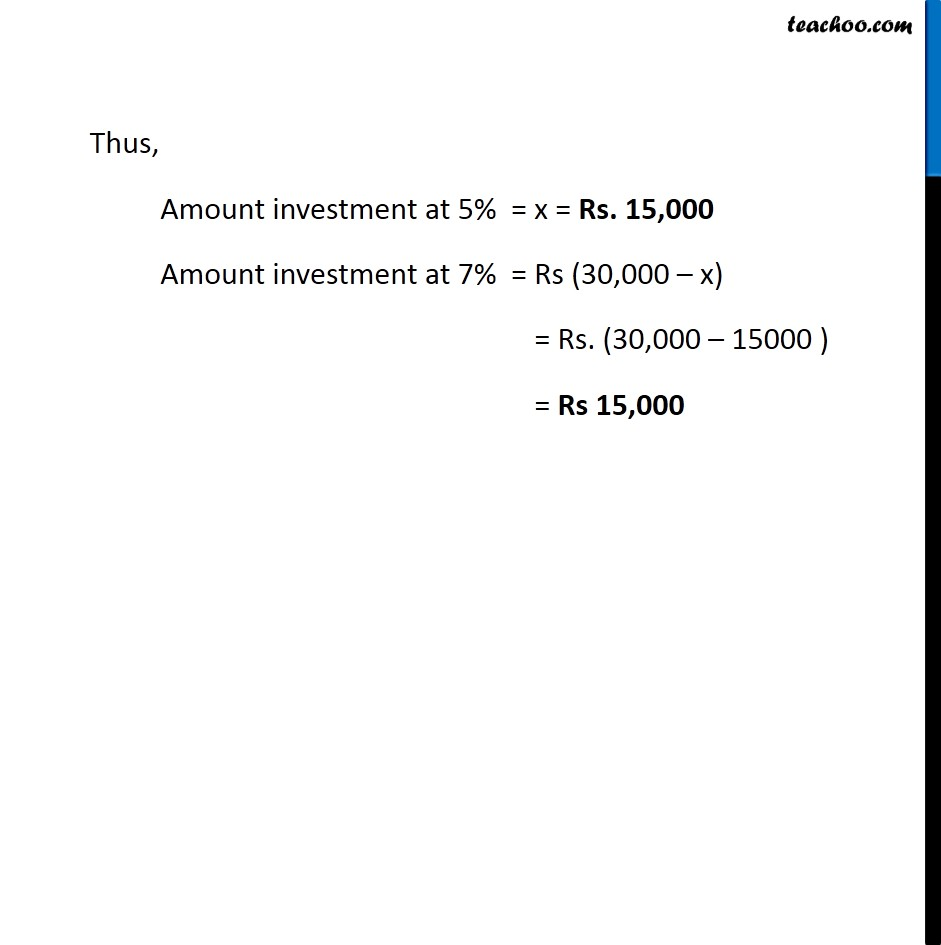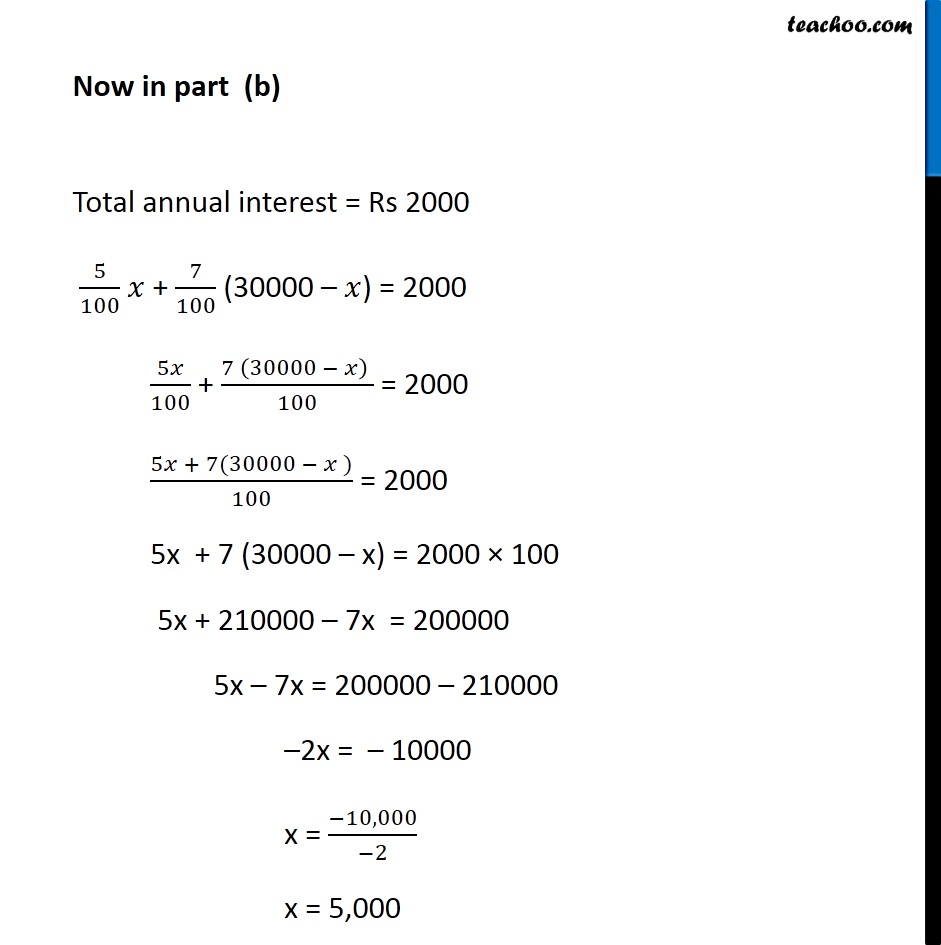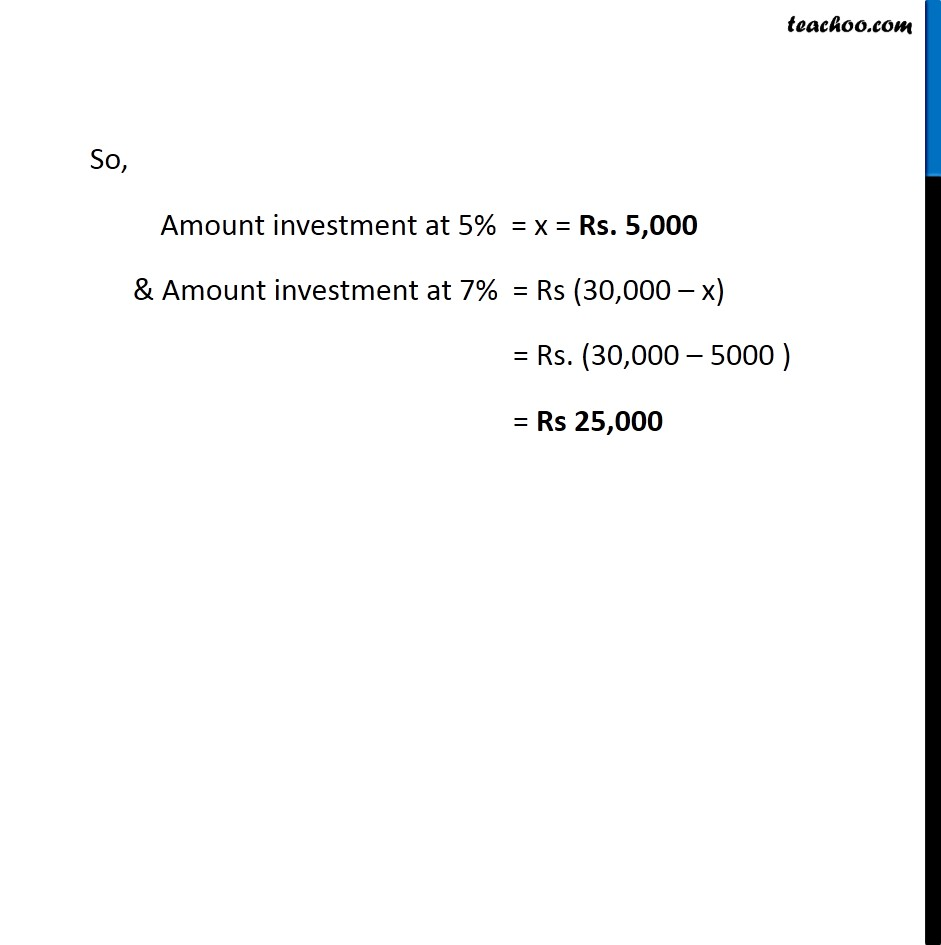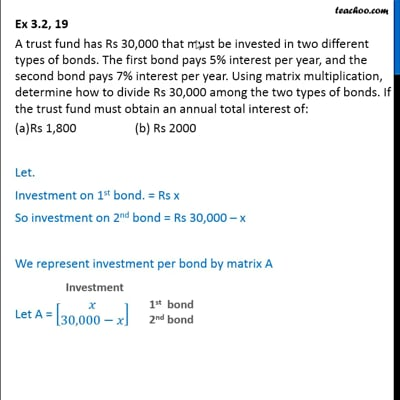This video is only available for Teachoo black users

Introducing your new favourite teacher - Teachoo Black, at only ₹83 per month

### Transcript

Ex 3.2, 19 A trust fund has Rs 30,000 that must be invested in two different types of bonds. The first bond pays 5% interest per year, and the second bond pays 7% interest per year. Using matrix multiplication, determine how to divide Rs 30,000 among the two types of bonds. If the trust fund must obtain an annual total interest of: Rs 1,800 (b) Rs 2000 Let Investment on 1st bond. = Rs x So, Investment on 2nd bond = Rs 30,000 – x We represent investment per bond by matrix A Let A = [■8(𝑥@30,000−𝑥)] Investment 1st bond The first bond pays 5% interest per year & The second bond pays 7% interest per year We represent interest per yer per bond by matrix B Let B = [■8(5%& 7%)] = [5/100 7/100] Now, Total Annual Interest = Interest per bond × Investment per bond = BA = [5/100 7/100]_(1 × 2) [■8(𝑥@30,000−𝑥)]_(2 ×1) = [5/100 𝑥" + " 7/100 " (30,000 –" 𝑥") " ]_(1 × 1) = 5/100 𝑥 + 7/100 (30000 – 𝑥) Interest on 1st bond Interest on 2nd bond Now in part (a) Total annual interest = Rs 1800 5/100 𝑥 + 7/100 (30000 – 𝑥) = 1800 5𝑥/100 + (7 (30000 − 𝑥) )/100 = 1800 (5𝑥 + 7(30000 − 𝑥 ))/100 = 1800 5x + 7 (30000 – x) = 1800 × 100 5x + 210000 – 7x = 180000 5x – 7x = 180000 – 210000 –2x = – 30000 x = (−30,000)/(−2) x = 15,000 Thus, Amount investment at 5% = x = Rs. 15,000 Amount investment at 7% = Rs (30,000 – x) = Rs. (30,000 – 15000 ) = Rs 15,000 Now in part (b) Total annual interest = Rs 2000 5/100 𝑥 + 7/100 (30000 – 𝑥) = 2000 5𝑥/100 + (7 (30000 − 𝑥) )/100 = 2000 (5𝑥 + 7(30000 − 𝑥 ))/100 = 2000 5x + 7 (30000 – x) = 2000 × 100 5x + 210000 – 7x = 200000 5x – 7x = 200000 – 210000 –2x = – 10000 x = (−10,000)/(−2) x = 5,000 So, Amount investment at 5% = x = Rs. 5,000 & Amount investment at 7% = Rs (30,000 – x) = Rs. (30,000 – 5000 ) = Rs 25,000